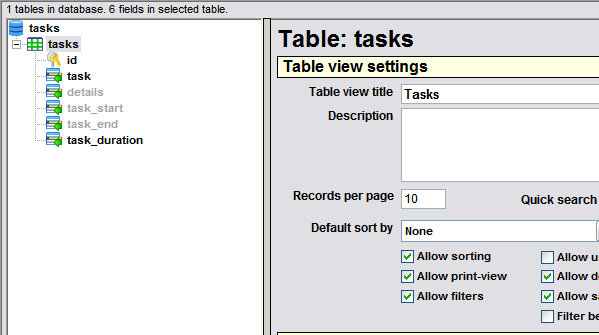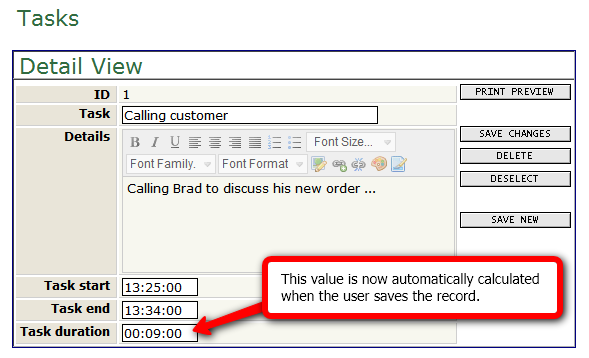Subscribe to AppGini tips and tutorials using your favorite RSS news reader by clicking the link to the right.
Read more AppGini tips and tutorials

# Automatically calculated fields

Note: This tutorial applies to AppGini versions prior to 4.50. For similar modifications applicable to AppGini 4.50 and higher, please use hooks. Please see the documentation and examples of the tablename_before_insert and tablename_before_update hooks.Let's say that you have a tasks table in your AppGini project. In this table, you have a task_start, a task_end, and a task_duration fields. You want your application to automatically calculate the value of the task_duration field from task_start and task_end. This tutorial shows you how to modify the generated code to achieve this.Assuming that our table is named tasks, we should find a file named tasks_dml.php among the AppGini-generated files. This file is where we need to add the code to automatically calculate the value of the task_duration field. So, let's open that file in a text editor, and find the line that begins with this code:

`\$task_duration = `

We should be able to find this line twice. Now, let's change both lines to read:

`\$task_duration = (\$task_start && \$task_end ? gmdate('H:i:s', strtotime(\$task_end)-strtotime(\$task_start)) : '');`

The above line of code does several things: it makes a simple check to see if both the task_start and task_end fields have a value, and if so calculates the difference in seconds and formats it as a time value. Based on the required calculation, your code might be simpler or more complicated than the above example. Here is the automatically calculated task duration after the above code modification.One last thing: In the above tutorial, we made the code modification in 2 places in the tasks_dml.php file. The first line is inside the tasks_insert() function and is applied when a new record is added. The second one is inside the tasks_update() function and is applied when an existing record is updated. So, if you want to make the automatic calculation only for new records, or only when existing records are updated, you should add your code only once in the desired place.

### More examples

• Here are some more examples of common formulas. Let's say you have a table containing these 3 fields: unit_price, quantity and total. You want to calculate the total using the unit_price and quantity fields. The formula would be:

`\$total = \$unit_price * \$quantity;`

• Another example: calculating the value of the total field using the fields subtotal, discount and sales_tax, where discount and sales_tax are stored as percentages (i.e. a discount value of 10 means 10% of subtotal):

``` \$total = \$subtotal * (1 - \$discount/100); // total after applying discount \$total = \$total * (1 + \$sales_tax / 100); // total after applying sales tax ```